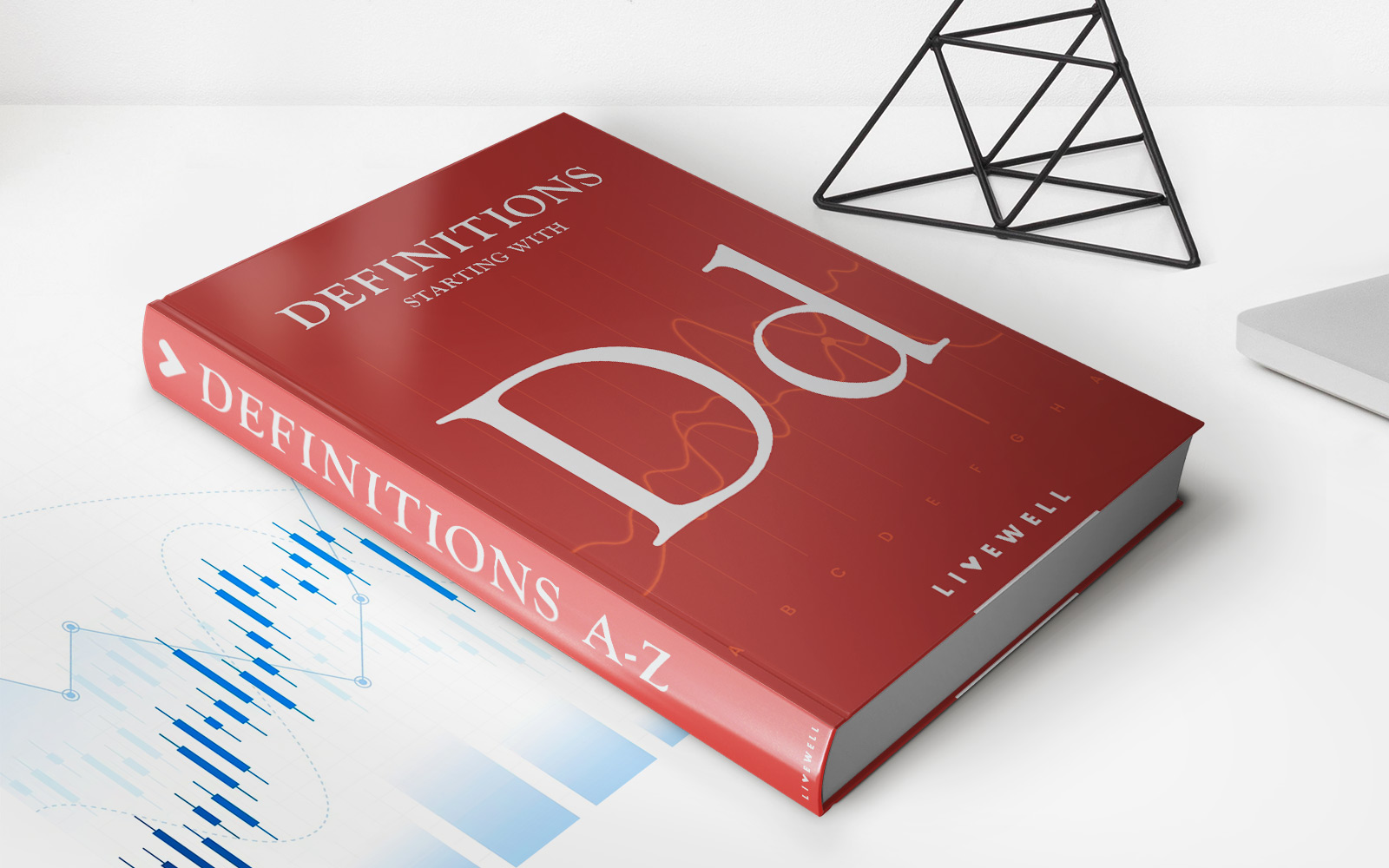Home>Finance>Debt-to-Capital Ratio: Definition, Formula, And ExampleFinance

# Debt-to-Capital Ratio: Definition, Formula, And Example

Learn the definition, formula, and example of the debt-to-capital ratio in finance. Understand how to calculate this vital financial metric.

## Welcome to the world of finance!

Today, we’re going to delve into a crucial financial metric known as the Debt-to-Capital Ratio. Have you ever wondered how companies determine their level of debt or how they manage their capital? Well, wonder no more! In this blog post, we’ll walk you through the definition, formula, and provide you with an example to illustrate its practical application. So, let’s get started on this financial journey!

## Key Takeaways:

• The Debt-to-Capital Ratio measures the proportion of a company’s debt to its total capital.
• It helps investors and lenders evaluate a company’s financial risk and solvency.

## What is the Debt-to-Capital Ratio?

The Debt-to-Capital Ratio is a financial metric used by investors, lenders, and analysts to assess a company’s financial structure. It measures the proportion of a company’s debt to its total capital, which includes both debt and equity. This ratio provides insight into a company’s ability to cover its financial obligations and the level of risk associated with its capital structure.

In simpler terms, the Debt-to-Capital Ratio indicates how reliant a company is on borrowed funds to finance its operations. Lenders and investors pay close attention to this ratio because it helps them assess the financial health and stability of a company. A high Debt-to-Capital Ratio may signify a company has a significant amount of debt and could be at risk of financial distress, while a low ratio indicates a more conservative financial structure.

## Calculating the Debt-to-Capital Ratio:

The formula for calculating the Debt-to-Capital Ratio is quite straightforward:

Debt-to-Capital Ratio = Total Debt / (Total Debt + Total Equity)

The Total Debt encompasses all outstanding debts a company owes, including short-term and long-term liabilities. On the other hand, the Total Equity represents the ownership interest in the company and can be found on the balance sheet.

Let’s take a look at an illustrative example:

Company XYZ has a total debt of \$2 million and total equity of \$8 million. By using the formula mentioned above, we can calculate their Debt-to-Capital Ratio:

Debt-to-Capital Ratio = \$2 million / (\$2 million + \$8 million) = \$2 million / \$10 million = 0.2 = 20%

So, Company XYZ has a Debt-to-Capital Ratio of 20%.

## Why is the Debt-to-Capital Ratio Important?

Now that we understand how to calculate the Debt-to-Capital Ratio, let’s discuss its importance. This ratio is essential because:

1. Assessing Financial Risk: A high Debt-to-Capital Ratio indicates a higher financial risk due to higher debt levels. This ratio helps investors and lenders evaluate the likelihood of a company defaulting on its obligations.
2. Comparative Analysis: Companies within the same industry can be compared using the Debt-to-Capital Ratio. This allows investors to identify companies with healthy financial structures and make well-informed investment decisions.

Additionally, the Debt-to-Capital Ratio contributes to a company’s credit rating and borrowing costs. Companies with lower ratios are often viewed as less risky borrowers, allowing them to borrow at lower interest rates.

## Conclusion

The Debt-to-Capital Ratio is a vital financial metric used by investors, lenders, and analysts to assess a company’s financial health and risk. By understanding this ratio and how to calculate it, you gain valuable insights into a company’s financial structure and the level of debt it carries. Remember, a lower ratio generally signifies a more stable financial position, while a higher ratio indicates higher risk. So, the next time you dive into the world of finance, remember to pay attention to the Debt-to-Capital Ratio!

• https://livewell.com/finance/debt-to-capital-ratio-definition-formula-and-example/